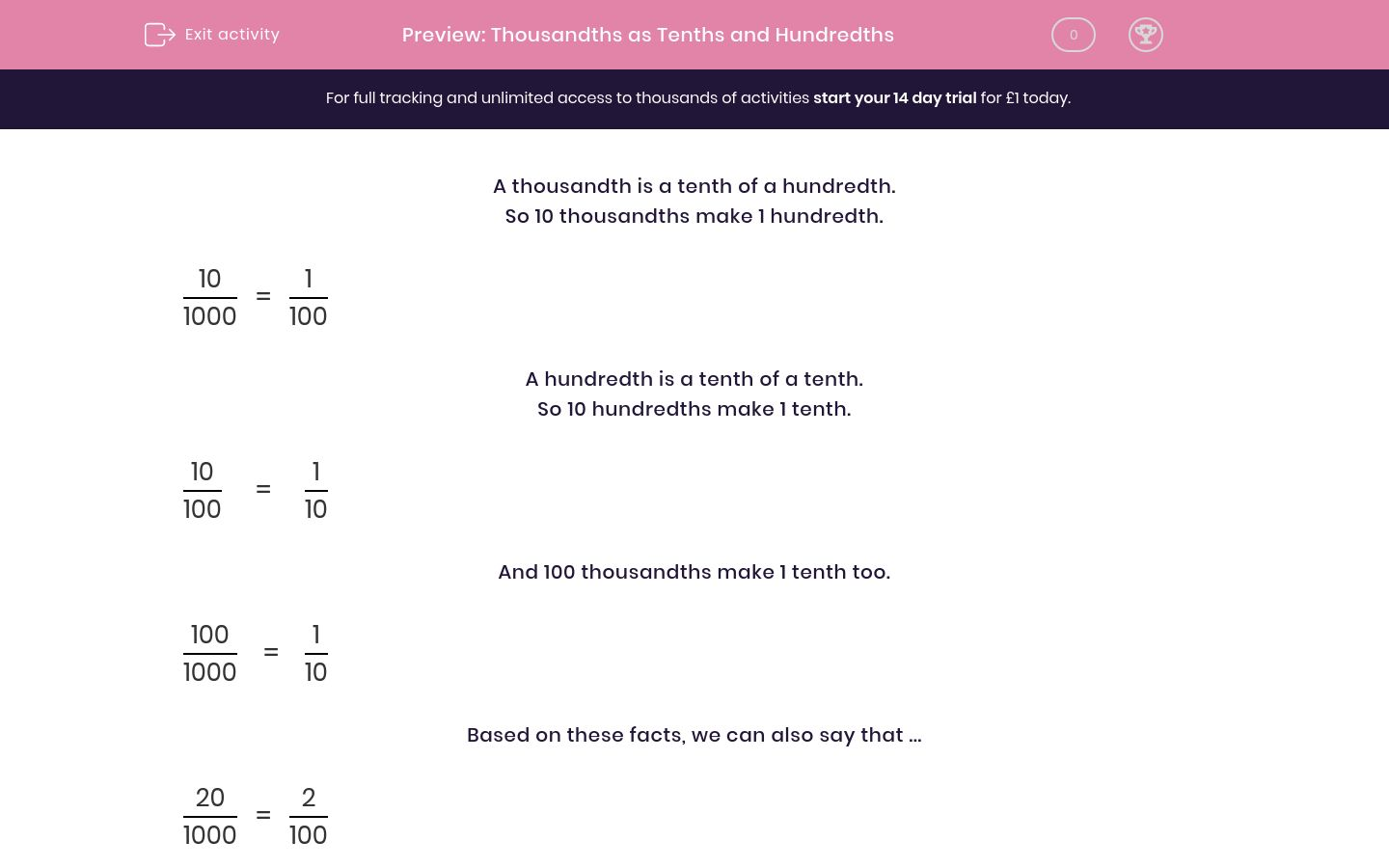# Thousandths as Tenths and Hundredths

In this worksheet, students recognise and use thousandths and relate them to tenths and hundredths.Key stage:  KS 2

Curriculum topic:   Maths and Numerical Reasoning

Curriculum subtopic:   Fractions

Difficulty level:### QUESTION 1 of 10

A thousandth is a tenth of a hundredth.

So 10 thousandths make 1 hundredth.

 10 = 1 1000 100

A hundredth is a tenth of a tenth.

So 10 hundredths make 1 tenth.

 10 = 1 100 10

And 100 thousandths make 1 tenth too.

 100 = 1 1000 10

Based on these facts, we can also say that ...

 20 = 2 1000 100

 30 = 3 100 10

 600 = 6 1000 10

True or false?

 10 = 1 1000 100
true

false

True or false?

 40 = 4 1000 100
true

false

True or false?

 70 = 7 1000 10
true

false

True or false?

 10 = 1 100 10
true

false

True or false?

 60 = 6 100 10
true

false

True or false?

 90 = 9 100 100
true

false

True or false?

 100 = 1 1000 10
true

false

True or false?

 200 = 2 1000 10
true

false

True or false?

 500 = 5 1000 10
true

false

True or false?

 900 = 9 1000 10
true

false

• Question 1

True or false?

 10 = 1 1000 100
true
EDDIE SAYS
10 thousandths make 1 hundredth.
• Question 2

True or false?

 40 = 4 1000 100
true
EDDIE SAYS
10 thousandths make 1 hundredth.
• Question 3

True or false?

 70 = 7 1000 10
false
EDDIE SAYS
10 thousandths make 1 hundredth.
70 thousandths make 7 hundredths.
• Question 4

True or false?

 10 = 1 100 10
true
EDDIE SAYS
10 hundredths make 1 tenth.
• Question 5

True or false?

 60 = 6 100 10
true
EDDIE SAYS
10 hundredths make 1 tenth.
• Question 6

True or false?

 90 = 9 100 100
false
EDDIE SAYS
10 hundredths make 1 tenth.
90 hundredths make 9 tenths.
• Question 7

True or false?

 100 = 1 1000 10
true
EDDIE SAYS
10 thousandths make 1 hundredth.
100 thousandths make 10 hundredths, which make 1 tenth.
• Question 8

True or false?

 200 = 2 1000 10
true
EDDIE SAYS
10 thousandths make 1 hundredth.
200 thousandths make 20 hundredths, which make 2 tenths.
• Question 9

True or false?

 500 = 5 1000 10
true
EDDIE SAYS
10 thousandths make 1 hundredth.
500 thousandths make 50 hundredths, which make 5 tenths.
• Question 10

True or false?

 900 = 9 1000 10
true
EDDIE SAYS
10 thousandths make 1 hundredth.
900 thousandths make 90 hundredths, which make 9 tenths.
---- OR ----

Sign up for a £1 trial so you can track and measure your child's progress on this activity.

### What is EdPlace?

We're your National Curriculum aligned online education content provider helping each child succeed in English, maths and science from year 1 to GCSE. With an EdPlace account you’ll be able to track and measure progress, helping each child achieve their best. We build confidence and attainment by personalising each child’s learning at a level that suits them.

Get started